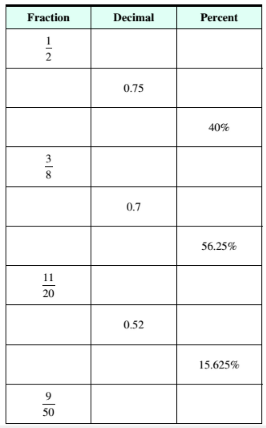Chapter 9.3, Problem 7ESMathematical Excursions (MindTap C...

4th Edition
Richard N. Aufmann + 3 others
ISBN: 9781305965584

Solutions

Chapter
SectionMathematical Excursions (MindTap C...

4th Edition
Richard N. Aufmann + 3 others
ISBN: 9781305965584
Textbook Problem

Complete the table of equivalent fractions, decimals, and percent’s.To determine

To calculate: The fraction and percent for the decimal 0.7 .

Explanation

Given Information:

Decimal, 0.7

Formula used:

Steps to write decimal as fraction,

Step I

: Calculate the percent value of the decimal.

Step II

: Remove the percent sign of the percentage value and multiply the number by 1100 .

Step III: Simplify the fraction.

Calculation:

Consider the decimal,

0.7

Move the decimal point two places to the right and place a percent sign, to write decimal as percent,

0.7=70%

Thus, the percent value of decimal 0.7 is 70% .

Now, remove the percent sign from the percentage value and multiply it by 1100

Still sussing out bartleby?

Check out a sample textbook solution.

See a sample solution

The Solution to Your Study Problems

Bartleby provides explanations to thousands of textbook problems written by our experts, many with advanced degrees!

Get Started

2. Define the terms population, sample, parameter and statistic.

Statistics for The Behavioral Sciences (MindTap Course List)

Convert the expressions in Exercises 6584 to power form. 3

Finite Mathematics and Applied Calculus (MindTap Course List)

Find the prime factorization of 696

Elementary Technical Mathematics

Identify the threats to internal validity for pre-post designs.

Research Methods for the Behavioral Sciences (MindTap Course List)

The general solution to (for x, y > 0) is: a) y = ln x + C b) c) y = ln(ln x + C) d)

Study Guide for Stewart's Single Variable Calculus: Early Transcendentals, 8th